#### 期刊菜单

Gravity, Gravitational Fields, and Gravitons—Inference about the Frequency of Gravitational Energy Waves
DOI: 10.12677/AAS.2022.101001, PDF, HTML, XML, 下载: 133  浏览: 557

Abstract: Through the analysis of resonance, this paper puts forward an inference about gravitation: gravitation is an energy wave with a frequency of 1.875 × 10^23 Hz, its frequency is higher than that of gamma rays, and it can penetrate any matter. The smallest unit of gravitation is proton and neutral Sub (nucleon), each proton and neutron (nucleon) unit (1 s) time can emit a maximum of 0.937 × 10^23 graviton, the diameter of the graviton is less than 10^−20 m, the gravitational force of the two planets is in proportion to the transferred energy of gravitons. The action distance of gravitation is limited, and the gravitational paradox does not hold.

1. 共振是宇宙间最普遍的自然现象

γ射线又称γ粒子流，是原子核能级跃迁退激时释放出的射线，是波长短于0.01埃的电磁波(1埃 = 10^−10 m)，能量高于1.24 MeV，频率超过300 EHz (3 × 10^20 Hz)。γ射线对细胞有杀伤力，医疗上用来治疗肿瘤。γ射线有很强的穿透力，工业中可用来探伤或流水线的自动控制。粒子加速器  对于物理学的研究和发展是至关重要的，而粒子加速器就是共振原理的运用。在粒子物理的基本小宇宙中，每一种能量都有对应的频率，反之亦然，这是很自然的物质互补原理，既有波又有粒子的特性。物质因为具有波的性质，也就有了频率。粒子加速器就是运用了这样的共振原理，把许多小小的“波纹”迭加起来，结果变成很大的“波峰”，可把电子或质子推到近乎光速，在高速的相撞下产生新的粒子。

2. 物质质量的基本单位是质子和中子

${\lambda }_{0}=1.6×{10}^{-15}\text{ }\text{ }\text{m}$ (1)

${f}_{0}=\frac{{V}_{C}}{{\lambda }_{0}}=\frac{3×{10}^{8}}{1.6×{10}^{-15}}=1.875×{10}^{23}\text{ }\text{ }\text{Hz}$ (2)

${T}_{0}=\frac{1}{{f}_{0}}=\frac{1}{1.875×{10}^{23}}=5.33×{10}^{-24}\text{ }\text{ }\text{s}$ (3)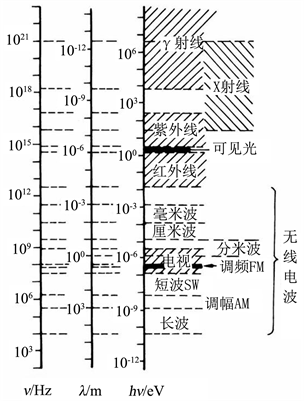Figure 1. Electromagnetic spectrum

3. 每个核子都可以发出引力子，形成引力能量场

$E=m{c}^{2}=1.67×{10}^{-27}×{\left(3×{10}^{8}\right)}^{2}=1.503×{10}^{-10}\text{ }\text{ }\text{J}$ (4)

${E}_{0}=hv=6.626×{10}^{-34}×1.875×{10}^{23}=1.242×{10}^{-10}\text{ }\text{ }\text{J}$ (5)

4. 处在引力矢量场中的核子与引力能量波产生共振，吸收引力子的能量，形成引力

5. 引力与引力子的关系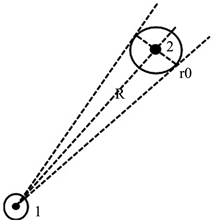Figure 2. Graviton transport

$\Delta E=G\frac{{M}_{1}{M}_{2}}{R}-G\frac{{M}_{1}{M}_{2}}{R+\Delta R}=G{M}_{1}{M}_{2}\left(\frac{1}{R}-\frac{1}{R+\Delta R}\right)=G{M}_{1}{M}_{2}\frac{\Delta R}{R\left(R+\Delta R\right)}$ (6)

$F\Delta R=G\frac{{M}_{1}{M}_{2}}{{R}^{2}}\Delta R=\Delta E=hN$ (7)

$F={k}_{0}N$ (8)

${k}_{0}=\frac{h}{\Delta R}=\frac{6.626×{10}^{-34}}{1.6×{10}^{-15-5}}=4.14×{10}^{-14}$ (9)

$\frac{1}{{k}_{0}}=\frac{1}{4.14×{10}^{-14}}=2.415×{10}^{13}$ (10)

$G\frac{{M}_{1}{M}_{2}}{{R}^{2}}={k}_{0}\frac{{M}_{1}}{{m}_{0}}\frac{{f}_{0}}{2}\frac{{r}_{0}^{2}}{{R}^{2}}{k}_{01}\frac{{M}_{2}}{{m}_{0}}\frac{{f}_{0}}{2}$ (11)

$G={k}_{0}{k}_{01}\frac{{f}_{0}^{2}{r}_{0}^{2}}{4{m}_{0}^{2}}$ (12)

${k}_{01}=\frac{4G{m}_{0}^{2}}{{k}_{0}{f}_{0}^{2}{r}_{0}^{2}}=\frac{4×6.67×{10}^{-11}×{\left(1.67×{10}^{-27}\right)}^{2}}{4.14×{10}^{-14}×{\left(1.875×{10}^{23}\right)}^{2}×{\left(0.8×{10}^{-15}\right)}^{2}}=7.988×{10}^{-67}$ (13)

${k}_{10}={k}_{20}=\sqrt{{k}_{01}}=\sqrt{7.988×{10}^{-67}}=8.94×{10}^{-34}$ (14)

${N}_{1\text{kg}}=\frac{1}{{m}_{0}}\frac{{f}_{0}}{2}{k}_{10}=\frac{1.875×{10}^{23}×8.94×{10}^{-34}}{2×1.67×{10}^{-27}}=5.02×{10}^{16}$ (15)

6. 引力作用距离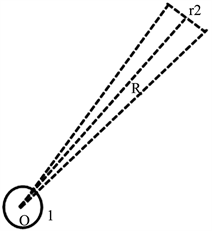Figure 3. Gravitational distance

$\frac{M}{{m}_{0}}\frac{{f}_{0}}{2}{k}_{10}\frac{{r}_{2}^{2}}{{R}^{2}}=\left(\frac{1}{3}\pi R{r}_{2}^{2}\rho -M\frac{{r}_{2}^{2}}{{R}^{2}}\right)\frac{1}{{m}_{0}}\frac{{f}_{0}}{2}{k}_{20}$ (16)

$R={\left(\frac{6M}{\pi \rho }\right)}^{1/3}$ (17)

$M=\frac{4}{3}\pi {R}_{银}^{3}\rho /{N}_{0}$ (18)

${R}_{\text{max}}={\left(\frac{6}{\pi \rho }\frac{4}{3}\pi {R}_{银}^{3}\rho \frac{1}{{N}_{0}}\right)}^{1/3}=\frac{2{R}_{银}}{{N}_{0}^{1/3}}=\frac{1×{10}^{5}}{{\left(2×{10}^{12}\right)}^{1/3}}=7.94\text{\hspace{0.17em}}\text{ly}$ (19)

7. 引力场中的能量转移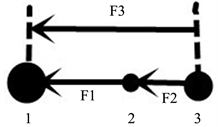Figure 4. Gravity weakens

8. 引力子大小

9. 夸克  的质量问题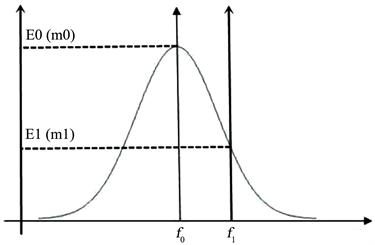Figure 5. Resonance energy curve

10. 引力悖论、本特利悖论、和西利格尔悖论   

11. 结论

  庄华梅, 何德. 核磁共振技术及其在生命科学中的应用[J]. 现代生物医学进展, 2005, 5(4): 58-61.  李际霖. 粒子加速器[J]. 物理通报, 1956(10): 557-584.  百度百科. 共振[EB/OL]. https://baike.baidu.com/item/%E5%85%B1%E6%8C%AF/1351785?fr=aladdin, 2022-03-27.  张嘉年. 原子核内部结构模型图表解析[J]. 科技与企业, 2015(8): 227-231.  罗旭东, 马红孺. 质能方程的一种简单推导方法[J]. 江西科学, 2005, 23(4): 418-420.  王莉, 朱浩, 孙燕云. 大学物理(下册) [M]. 北京: 清华大学出版社, 2018: 212-213.  王莉, 朱浩, 孙燕云. 大学物理(上册) [M]. 北京: 清华大学出版社, 2018: 57-60.  苏宜. 天文学新概论[M]. 第5版. 北京: 科学出版社, 2019: 91-92.  季灏. 宇宙年龄和宇宙物质密度[J]. 前沿科学, 2014, 8(4): 71-77.  苏宜. 天文学新概论[M]. 第5版. 北京: 科学出版社, 2019: 40-42, 519.  苏宜. 天文学新概论[M]. 第5版. 北京: 科学出版社, 2019: 108-117.  王莉, 朱浩, 孙燕云. 大学物理(下册) [M]. 北京: 清华大学出版社, 2018: 227-228.  百度百科. 中子[EB/OL]. https://baike.baidu.com/item/%E4%B8%AD%E5%AD%90/466557?fr=aladdin, 2022-03-27.  百度百科. 引力子[EB/OL]. https://baike.baidu.com/item/%E5%BC%95%E5%8A%9B%E5%AD%90/2190759?fr=aladdin, 2022-03-27.  百度百科. 量子引力理论[EB/OL]. https://baike.baidu.com/item/%E9%87%8F%E5%AD%90%E5%BC%95%E5%8A%9B%E7%90%86%E8%AE%BA/793833?fr=aladdin, 2022-03-27.  黄涛. 夸克30年[J]. 现代物理知识, 1995, 6(1): 2-5.  Norton, J.D. (1992) A Paradox in Newtonian Gravitation Theory. Proceedings of the Biennial Meeting of the Philosophy of Science Associa-tion, 1992, 412-420. https://doi.org/10.1086/psaprocbienmeetp.1992.2.192853  Norton, J.D. (2002) A Para-dox in Newtonian Gravitation Theory II. In: Meheus, J., Ed., Inconsistency in Science, Springer, Dordrecht, 185-195. https://doi.org/10.1007/978-94-017-0085-6_11  Horedt, G.P. (1989) On Seeliger’s Paradox. Astrophysics and Space Science, 159, 169-171. https://doi.org/10.1007/BF00640500